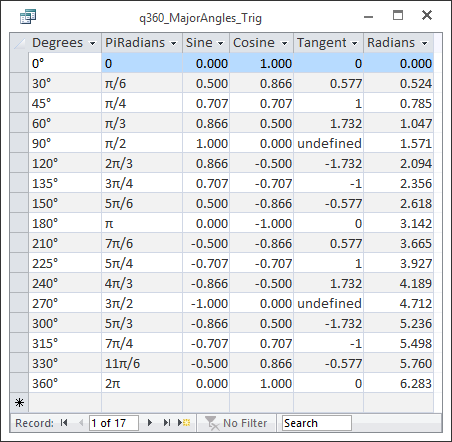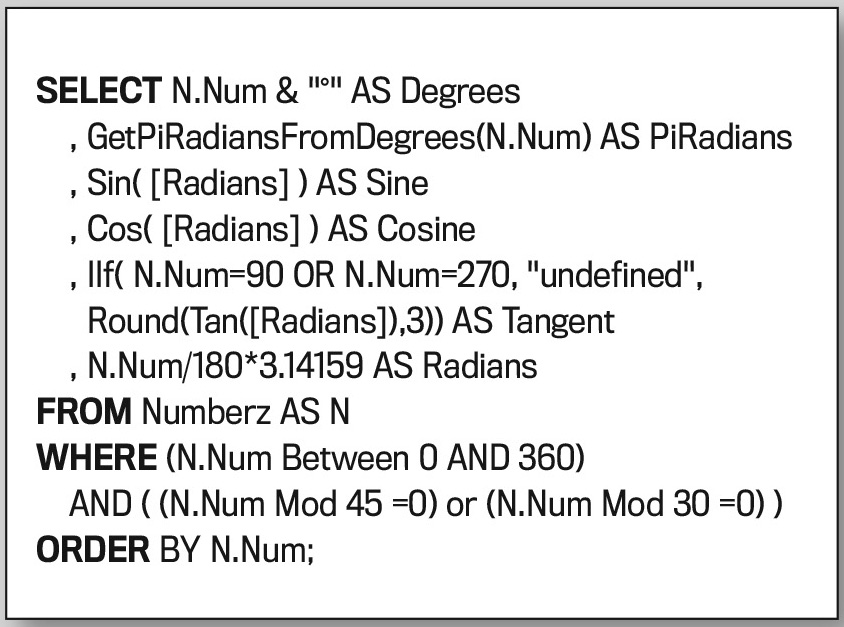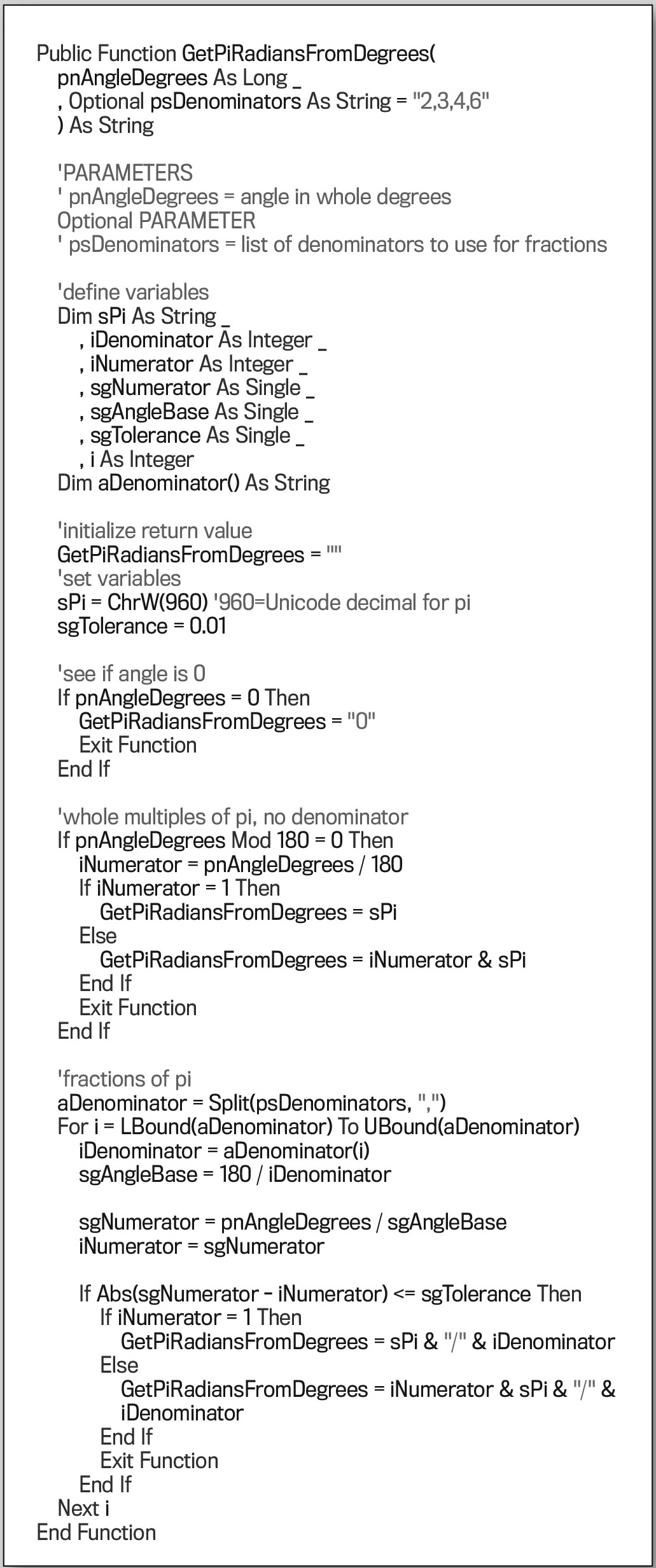### UNIT CIRCLE HANDOUT

Our hypothetical math teacher who is using Access to keep track of students and grades discovered that Access is also a great tool for making a Unit Circle handout that lists angles and trigonometric values, such as sine and cosine. Figure 1 shows a portion of that handout, which is the Datasheet view of a query with degrees for the major angles around a circle, radians, sine, cosine, tangent, and the angle expressed as a fraction in terms of pi. It’s based on a table called “Numberz” and uses a field called “Num” that contains sequential numbers.The SQL for the query is in Figure 2. The FROM clause indicates that the records for this query come from the Numberz table, which will be referred to as “N” throughout. The only field we actually use from the table is “Num.” The WHERE clause indicates that numbers from 0 to 360 that are evenly divisible by 45 or 30 will be used. The Mod operator returns the remainder of the first number divided by the second number. This will eliminate numbers like 37 and 99 since both would return a remainder that isn’t 0 when divided by 45 or by 30. The ORDER clause specifies ascending order by number.The SELECT clause specifies the list of fields to show. In this case, all of them are calculated fields. The first column is the number followed by a degree symbol (°) and will be called “Degrees.” You can get the degree symbol by typing ALT+248 on the numeric keypad.

The second column uses a custom function called GetPiRadiansFromDegrees to return a string representing the angle in radians, expressed as fractions of pi, given an angle in degrees.

The last field in the list, “Radians,” is a calculated field that converts degrees to radians by dividing the number representing an angle by 180 and multiplying it by pi.

Access already has the Sin, Cos, and Tan functions to calculate sine, cosine, and tangent, respectively. Each of these functions needs a parameter with the angle in radians. For that, we can refer to our calculated field with Radians.

The problem with tangent, however, is that it isn’t defined for 90° or 270°, and nonsense values are returned for those angles. Therefore, N.Num is tested to see if it’s value is 90 and 270. If it is, then the word “undefined” is displayed. Otherwise the tangent is calculated.

To set the number of decimal places to three, a format code of 0.000 was specified on the property sheet for each of the numeric columns. If you forget to do this, you’ll see numbers with lots of decimal places.

### A USER-DEFINED FUNCTION

There isn’t a built-in function we could use to show radians expressed in terms of pi. The solution is to write a user-defined function (UDF) to return the text that we want to see.

GetPiRadiansFromDegrees is defined as a public function in a standard module so it can be used anywhere in the database. It converts degrees to radians and returns a string specifying the mathematical text with pi or fractions of pi, like π/2, π/3, π/4, and π/6.

The first, and only required, parameter is called “pnAngleDegrees.” This represents the angle in degrees, and it’s a whole number. Optionally, you may specify a second parameter, “psDenominators,” with a string of acceptable denominators delimited with a comma. By default, this is 2,3,4,6.

This function takes advantage of the fact that we can use a Unicode character for pi, so we can construct a string that includes Greek characters. Figure 3 contains the VBA code.In the GetPiRadiansFromDegrees function, first variables are dimensioned. The string representation for the pi symbol (π) is assigned to sPi. The denominator is stored as a whole number in iDenominator. When the numerator is a whole number, it’s stored in iNumerator. When it has decimal places, it’s stored in sgNumerator. The base angle, sgAngleBase, is whatever you are dividing by to test a denominator, such as 30° or 45°. The tolerance, sgTolerance, is the maximum allowable difference to consider two numbers to be the same. It’s stored as a single precision number so it can have decimal places. Floating point numbers are stored in scientific notation and aren’t precise, so there must be some kind of tolerance to compare them.

The function will loop, so we’ll use a variable called i to keep track of which iteration we’re on. A string array called aDenominator is dimensioned to handle individual values in the passed string of acceptable denominators. Even though it contains numbers, it’s defined as a string so that the Split function can be used to populate its values.

Once the variables are defined, the return value of the function is initialized to be an empty string ("") in case the angle isn’t a whole fraction of pi.

Next, the variables are set. The Unicode character for pi (sPi) is assigned by specifying 960 as the decimal code for the built-in ChrW function. And sgTolerance is set to 0.01. This will be plenty of tolerance since angles are in whole degrees.

If the passed angle is 0, the pi symbol won’t be in the return string. In that case, the function return is set to 0, and the function exits.

Next, we test for angles that are whole multiples of pi. These instances won’t have a denominator. Pi is 180°. The Mod operator returns the remainder of the first number divided by the second. If the mod is 0, the mathematical string is calculated, and the function exits. The numerator is the angle in degrees divided by 180. If that’s equal to 1, only pi will be assigned to the return string. Otherwise, it will be the numerator followed by the symbol for pi.

If we’re still in the function at this point, then the angle wasn’t a whole multiple of pi.

So the next step is to test if the angle is an even fraction of pi given the list of denominators we want to display. The Split function is used on the string list in psDenominators to into an array of elements. Each element can be referenced as aDenominator(i), where i is the element number. The LBound and Ubound functions return the lower and upper element numbers of an array so we can iterate through it, and we use i as a counter. The aDenominator(i) element is assigned to iDenominator to turn it into an integer. The first time through, it’s value will be 2. The base angle, sgAngleBase, is what we’ll divide our angle by to get a fraction. It is 180 divided by the denominator being tested. The first time through the loop, the base angle will be 90.

The numerator is our passed angle divided by the base angle we’re dividing by in this iteration. That result, with decimal places, is sgNumerator, and it’s iNumerator as a whole number. If the absolute value of the difference between the numerator with and without decimal places is within the tolerance, the return string is constructed as before, adding "/" and the denominator to the end. If a return is calculated, the function exits. If not, the loop continues until all the denominators have been tested.

If no return string was calculated, the function will exit after the For loop at the End Function statement. It will return the value it was initialized to—an empty string.